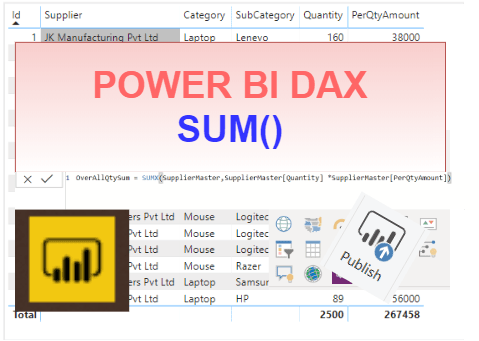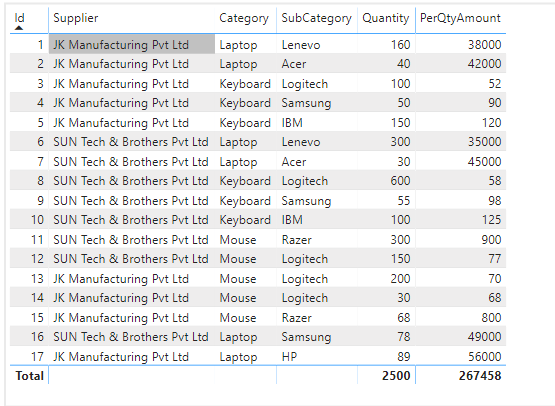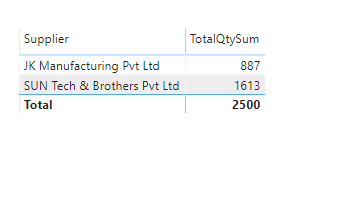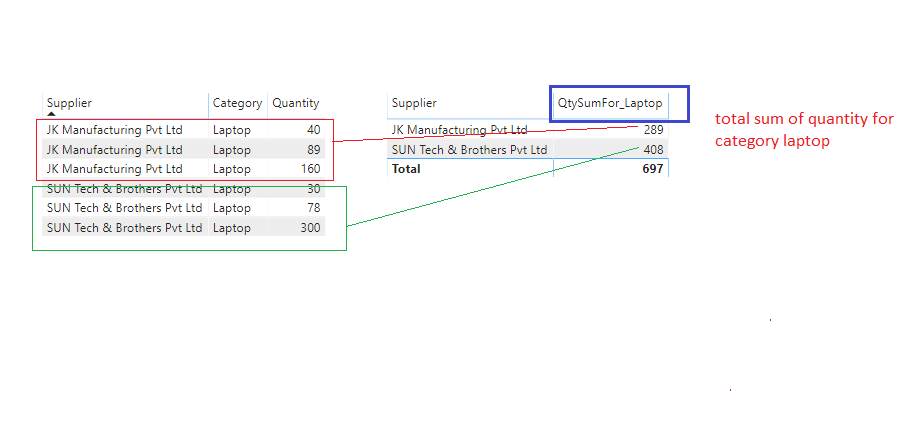Home » SUM() Function DAX

# SUM() Function DAX### SUM() function is a power bi dax mathematical function that adds up all the values in a single column that you  specify after applying all filters.

It can not perform row by row evaluation like SUMX() function.

It returns a decimal number.

DAX SYNTAX

SUM(<column>)

<column> is a column that contains the numbers to sum.

Lets go through an example to see SUM() function in action.

Here we have a sample table SupplierMaster which contains the data supplied by supplier as given below.Suppose you want display the total qunaity supplied by individual supplier so get the total qunatity can use SUM() function.

Lets create a measure to calculate the total sum of quantity

`TotalQtySum = SUM(SupplierMaster[Quantity])`As you can see, it returns a sum of total quantity for individaul supplier.Sum with CALCULATE Function

Suppose you want to display sum of quantity only for a specific category,  Lets say to calcualte the quantity sum for category  “Laptop” then you need to filter the data for category then calcualte the sum of quantity, in this case you can use CALULATE function as given below.

```QtySumFor_Laptop =
CALCULATE (
SUM ( SupplierMaster[Quantity] ),
SupplierMaster[Category] = "Laptop"
)```After creating a measure, you can see the output of this , a left table visual that displaying a supplier – category wise quantity for “Laptop” category and right table visual displaying the total quantity sum for category “Laptop” for individual suppliers.